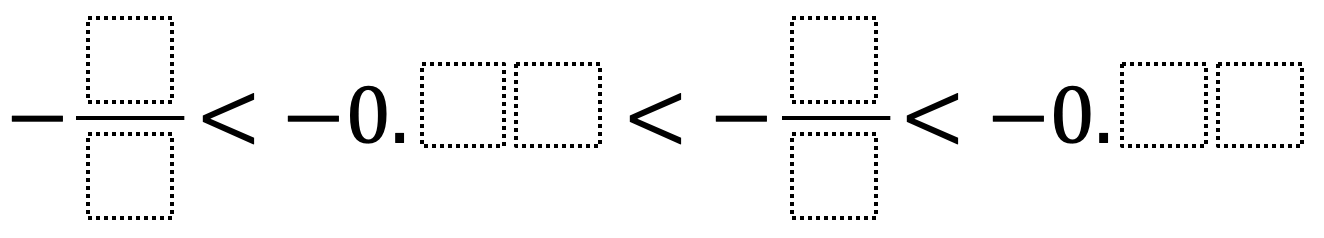# Ordering Rational Numbers

Directions: Using the digits 1 to 9 at most once each, fill in the boxes to make a true statement.### Hint

What importance does the relationship between the numerator and denominator have in determining the value of a fraction? What are some strategies to compare fractions and decimals?

There are various answers. A possible answer: -9/8 < -0.76 < -2/5 < -0.13 or -9/2 < -0.83 < -4/6 < -0.15

Source: Robert Millett

## Greatest Common Factor

Directions: Using the digits 0 to 9 at most one time each, place a digit …

1.A possible answer: -9/8 < -0.76 < -2/5 < -0.13 or -9/2 < -0.83 < -4/6 < -0.15

2.A possible answer to is -8/4 < -0.86 < – 3/4 < -0.50

3.A possible answer is -8/7 < -0.75 < -3/4 < – 0.12 < -8/2 < -0.82 < -3/6 < -0.14

4.5.Unknow is really me Brice but you still cheated Angel.

6.A possible answer to is -8/4 < -0.86 < – 3/4 < -0.50

7.A possible answer to is -5/2 < -0.46 < – 2/4 < -0.59

8.a possible is also 1/2 < -0.45 <-6/7<-0.89